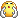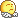#www.vustudents.ning.com

We non-commercial site working hard since 2009 to facilitate learning Read More. We can't keep up without your support. Donate.

 Assignment Question No. 1                                                                                                                                            In Bezier Curve if we want to add more control points between the starting and ending point of the Bezier Curve, then discuss with the help of the example, how shape of the Bezier Curve would be changed.                                                                                          Question No. 2                                                                                                                                              How can we express the third degree  Bezier Curve in matrix form, also write the parametric equation of  Bezier Curve.     You can send email at cs602@vu.edu.pk for any assignment related problem.   Your work must be original. No marks will be given in case of cheating or copying from the internet, handouts or from any other student and strict action will be taken against that student.

Views: 2062

Attachments:

### Replies to This Discussion

plzzz question ka ans yaha post kr dain smaj nahi a rahi :(..... Question 2 k leye thanks saqib bhai plzz Q1 ka b post kr dy smaj nahi a rahi jaisy ap bata rahy hain :(

kindly p0st idea solution :/

Send plzzzzzzzzzzzz assignment solution plzz plzzzplz send solution file plz

Pehlay b meri 2 assignment me se zero makrs aaye hain....ab ye to koi send kar do....

kisi nu kosh v samj lagi?

its very clear and simple.. first is basic eq and after we are adding one by one a control point and our eq changed and also cure shape

sb itna in secure q feel krty hen ab assignmnt solution upload krty :( men c0py nh krun ge paka wala ALLAH wala pr0mise idea solution t0u complete upl0ad kr do bx idea he lyn gy :(

If we add more control point between the starting and ending point our parametric equation for the cure will changed and according to the equation the shape of the curve will be changed.

This is our baisic equation.

x = fx(t) = (1 – t) ax + t·bx

y = gy(t) = (1 – t) ay + t·by

if we add one control point our equation become 2nd gedree equation and our equation shape of the curve will be ..

 fx(t) = (1 – t)2 ax + 2t(1 – t) bx + t2cx gy(t) = (1 – t)2 ay + 2t(1 – t) by + t2cy

P1  .

P0  .                     . P2

After adding one more control point our equation will become cubic equation and our curve will also be a cubic curve..

 fx(t) = (1-t)3 ax + 3t(1-t)2bx + 3t2(1-t) cx + t3dx gy(t) = (1-t)3ay + 3t(1-t)2by + 3t2(1-t) cy + t3dy

P1  .

P0  .                                                    . P3

. P2

ab is me curves nai post hoten wo khud bna len jesi oper picture me de hui hen